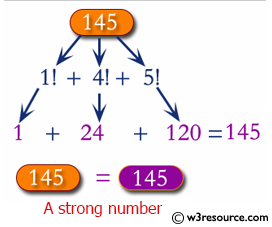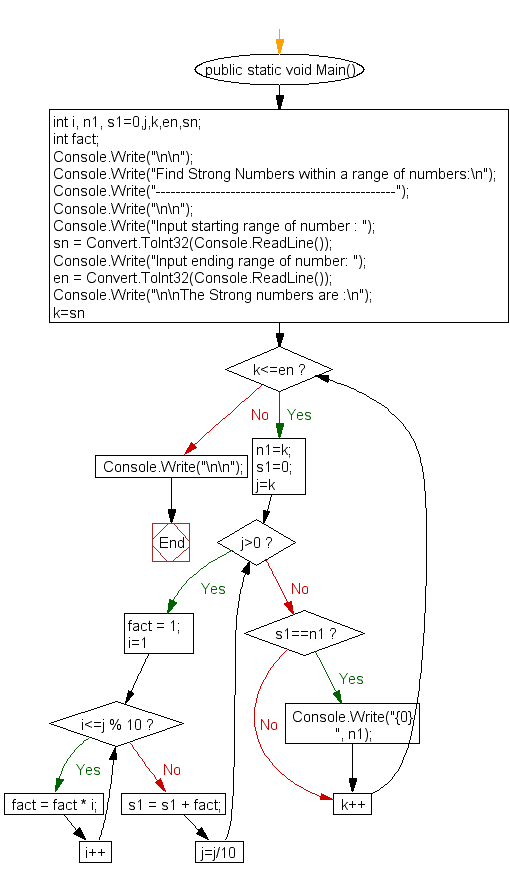﻿ C# - Check whether a number is Strong Number or not# C# Sharp Exercises: Check whether a number is Strong Number or not

## C# Sharp For Loop: Exercise-47 with Solution

Write a C# Sharp program to check whether a number is Strong Number or not.Sample Solution:-

C# Sharp Code:

``````using System;
public class Exercise47
{
public static void Main()
{
int i, n, n1, s1=0,j;
int fact;

Console.Write("\n\n");
Console.Write("Check whether a number is Strong Number or not:\n");
Console.Write("-------------------------------------------------");
Console.Write("\n\n");

/* If sum of factorial of digits is equal to original number then it is Strong number */

Console.Write("Input a number to check whether it is Strong number: ");
n1 = n;

for(j=n;j>0;j=j/10)
{

fact = 1;
for(i=1; i<=j % 10; i++)
{
fact = fact * i;
}
s1 = s1 + fact;

}

if(s1==n1)
{
Console.Write("\n{0} is Strong number.\n\n", n1);
}
else
{
Console.Write("\n{0} is not Strong number.\n\n", n1);
}
}
}
```
```

Sample Output:

```Check whether a number is Strong Number or not:
-------------------------------------------------
Input a number to check whether it is Strong number: 2
2 is Strong number.
```

Flowchart:C# Sharp Code Editor:

Contribute your code and comments through Disqus.

What is the difficulty level of this exercise?

Test your Programming skills with w3resource's quiz.

﻿